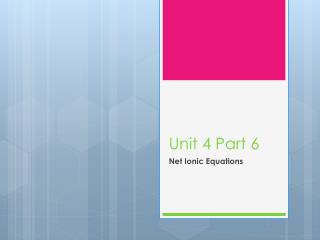DownloadDownload PresentationUnit 4 Part 6

# Unit 4 Part 6

Download Presentation## Unit 4 Part 6

- - - - - - - - - - - - - - - - - - - - - - - - - - - E N D - - - - - - - - - - - - - - - - - - - - - - - - - - -
##### Presentation Transcript

1. Unit 4 Part 6 Net Ionic Equations

2. Balancing Net Ionic Equations: • Before learning how to write balanced net ionic equations, we must first learn about electrolytes.

3. Electrolytes • If an aqueous solution of a compound conducts electricity, it is called an electrolyte. • The ability to conduct electricity results when an aqueous solution contains dissolved ions. There are two types of electrolytes:

4. Electrolytes • Strong Electrolytes - These compounds are the ones that completely dissociate and exist as ions in solutions. They include strong acids (HCl, HBr, HI, HNO3, H2SO4 and HClO4, etc.), strong bases (Hydroxides etc.) and all soluble salts. • Weak Electrolytes - in aqueous solution, these compounds yield some ions in solution. They include: weak acids (any acid that isn't strong), and weak bases (any base that isn't strong). • Non-electrolytes – These are solutions that do not dissociate into ions. This includes water.

5. Balancing Net Ionic: • Net ionic equations are simplified equations that represent chemical reactions with aqueous solutions involved. • Aqueous solutions are solutions created by salts dissolving in water, and their ions dissociating. • Solids, liquids and gases do not dissociate into ions. They stay in their compound or formula unit form.

6. There are 3 steps to writing out a Balanced Net Ionic Equation. • Step 1: Write a Balanced General Equation. • Step 2: Write the Total Ionic Equation. This means to look at each product and reactant and determine if it dissociates into its ions. If it does, you must write out these ions. • Step 3: Write the net ionic equation. That is to remove all ions that do not undergo a change. These are called spectator ions and these can be identified if they appear as ions in both the products and the reactants.

7. Ex. NaOH(aq) + HCl(aq) H2O(l) + NaCl(aq)

8. When writing net ionic equations we separate anything that is aqueous into its ions. Anything that is solid, liquid or gas stays as it is.

9. Examples: • KCl(aq) + AgNO3(aq)AgCl(s) + KNO3(aq) Total ionic equation Net ionic equation

10. Examples: NaOH(aq) + CuCl2(aq) Cu(OH)2(s) + NaCl(aq)

11. Not only do the atoms/elements need to balance but charges must also ALWAYS balance. If they do not then there is an error.

12. Bringing it all together: • Using reaction types to predict the products and solubility to write a balanced net ionic equation • Lead (II) Nitrate and Potassium Chloride

13. Assignments: • Pg 28 odd & 29 all